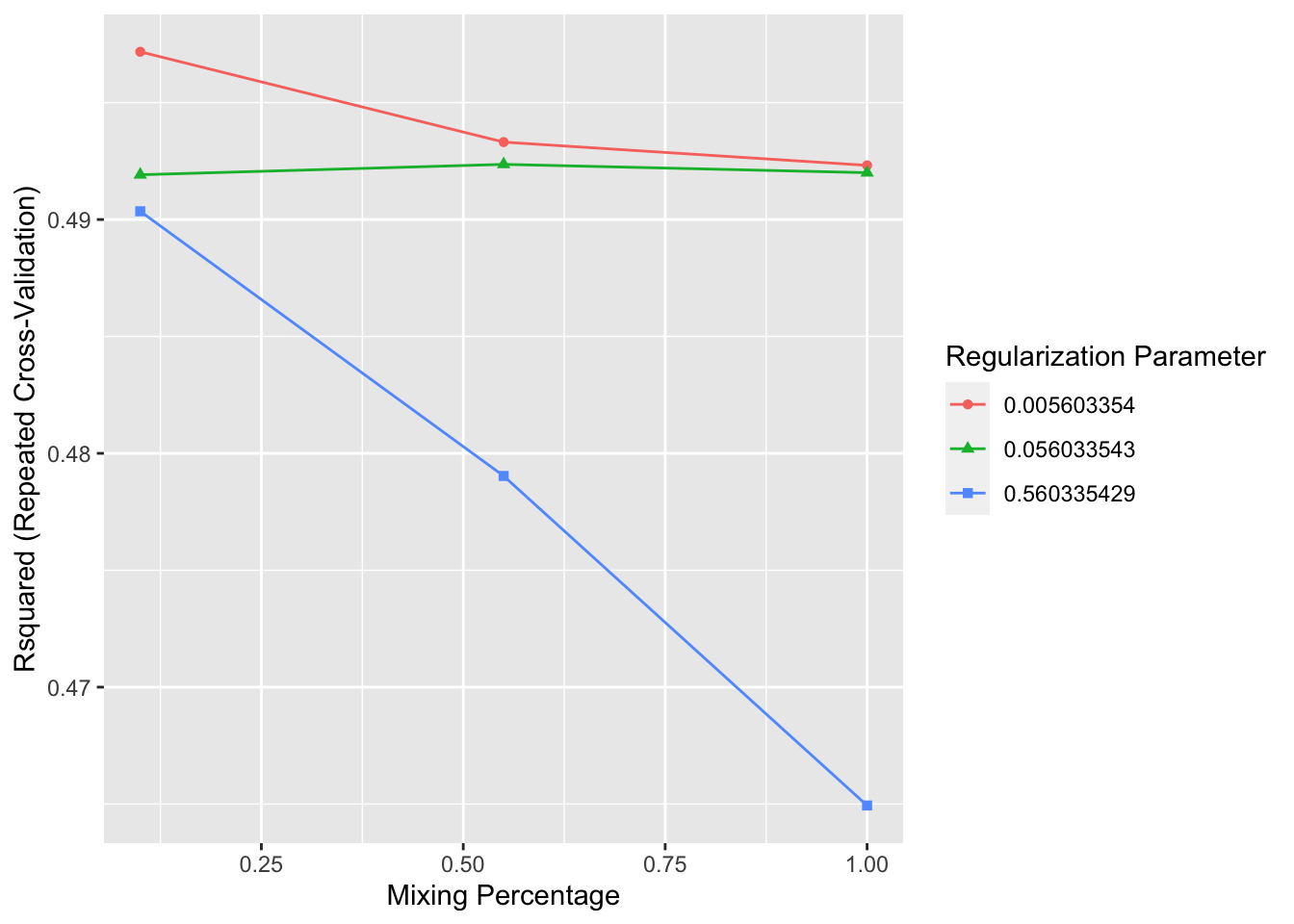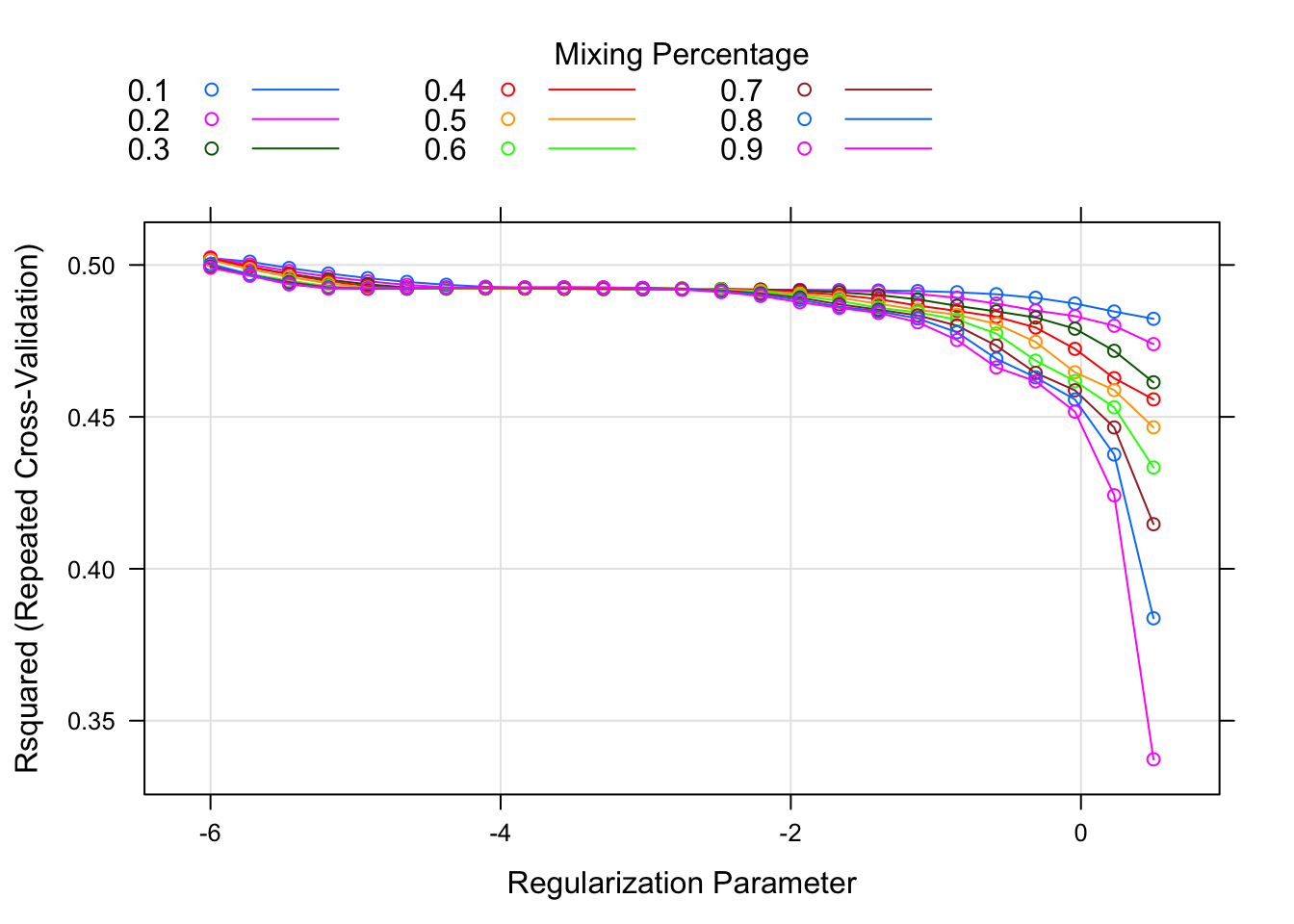library(tidyverse)
## ── Attaching packages ─────────────────────────────────────────────────────────────────────────────────────────────────────────────────────── tidyverse 1.3.0 ──
## ✓ ggplot2 3.3.2     ✓ purrr   0.3.4
## ✓ tibble  3.0.3     ✓ dplyr   1.0.2
## ✓ tidyr   1.1.1     ✓ stringr 1.4.0
## ✓ readr   1.3.1     ✓ forcats 0.5.0
## ── Conflicts ────────────────────────────────────────────────────────────────────────────────────────────────────────────────────────── tidyverse_conflicts() ──
## x dplyr::lag()    masks stats::lag()
df <- readr::read_rds("df.rds")
mod_lm_step1 <- readr::read_rds("mod_lm_step1.rds")

## Overview

1. Exploration
2. Regression models
3. Binary classification option b
4. Binary classification option a
5. Interpretation and optimization

This R Markdown file tackles part iiC, specifically training, evaluating, tuning, and comparing models for response_1 to the step 1 inputs, using more complex methods, via resampling.

iiA. Regression models - lm() iiB. Regression models - Bayesian iiC. Regression models - Models with Resampling

We will use the caret package to handle training, testing, and evaluation.

library(caret)
## Loading required package: lattice
##
## Attaching package: 'caret'
## The following object is masked from 'package:purrr':
##
##     lift

## General

Throughout all methods, we will use 5-fold cross validation, as our resampling method, by specifying "repeatedcv" as the method argument to a caret::trainControl(). Similar to before, we will use Rsquared as our performance metric.

From here on out, we will use basis_form to refer to our previously defined basis formula.

my_ctrl <- caret::trainControl(method = "repeatedcv", number = 5, repeats = 5)
rsquared_metric <- "Rsquared"
basis_form <- as.formula(paste("response_1 ~ xA + xB*(splines::ns(x01, df = 2) + splines::ns(x02, df = 2) + splines::ns(x03, df = 2))"))

First we will train a linear additive model on our previously defined basis function using caret::train and setting method="lm".

The main purpose of this linear additive model is to provide a baseline comparison to the other complex models we will train.

set.seed(12345)
method = "lm",
metric = rsquared_metric,
trControl = my_ctrl,
data = step_1_df)

mod_lin_add
## Linear Regression
##
## 2013 samples
##    5 predictor
##
## No pre-processing
## Resampling: Cross-Validated (5 fold, repeated 5 times)
## Summary of sample sizes: 1611, 1609, 1611, 1610, 1611, 1610, ...
## Resampling results:
##
##   RMSE      Rsquared   MAE
##   1.829145  0.8857109  1.439504
##
## Tuning parameter 'intercept' was held constant at a value of TRUE

## Regularized regression with elastic net

We will train two different models with interactions, specifically one with all pair interactions between all step 1 input variables, and one with all triplet interactions. Given $$n$$ coefficients, the number of coefficients that must be learned for a model with all $$m$$-input interactions will be modeled by:

$\sum_{k=1}^{m} \left( \begin{array} {c} n \\ k \end{array} \right)$

However, for each categorical input variable, we have to subtract the number of possibilites of interactions between categories within a single variable.

So, for all pair interactions between all step 1 continuous input variables, the number of coefficients that must be learned is $$10 + \left(\begin{array}{c} 10 \\ 2 \end{array}\right) - \left(\begin{array}{c} 3 \\ 2 \end{array}\right) = 52$$, excluding intercept. For all triplet interactions, the number of coefficients that must be learned is $$52 + \left(\begin{array}{c} 10 \\ 3 \end{array}\right) - 3\left(\begin{array}{c} 10 - 3 \\ 1 \end{array}\right) - \left(\begin{array}{c} 3 \\ 3 \end{array}\right) = 150$$, excluding intercept.

### All pair interactions between step 1 inputs

Let’s first fit a regularized regression model with elastic net, on all pairwise interactions between all step 1 inputs, using caret::train with method="glmnet". We specify centering and scaling as preprocessing steps.

set.seed(12345)
mod_glmnet_2 <- caret::train(response_1 ~ (.)^2,
method = "glmnet",
preProcess = c("center", "scale"),
metric = rsquared_metric,
trControl = my_ctrl,
data = step_1_df)

mod_glmnet_2
## glmnet
##
## 2013 samples
##    8 predictor
##
## Pre-processing: centered (52), scaled (52)
## Resampling: Cross-Validated (5 fold, repeated 5 times)
## Summary of sample sizes: 1611, 1609, 1611, 1610, 1611, 1610, ...
## Resampling results across tuning parameters:
##
##   alpha  lambda       RMSE      Rsquared   MAE
##   0.10   0.005603354  3.834367  0.4971762  2.775565
##   0.10   0.056033543  3.854567  0.4919151  2.784784
##   0.10   0.560335429  3.866934  0.4903532  2.771874
##   0.55   0.005603354  3.849215  0.4933127  2.784406
##   0.55   0.056033543  3.853397  0.4923624  2.778033
##   0.55   0.560335429  3.951536  0.4790317  2.805659
##   1.00   0.005603354  3.853070  0.4923175  2.785426
##   1.00   0.056033543  3.855883  0.4920035  2.775085
##   1.00   0.560335429  4.064377  0.4649381  2.878238
##
## Rsquared was used to select the optimal model using the largest value.
## The final values used for the model were alpha = 0.1 and lambda = 0.005603354.

Check number of coefficients:

mod_glmnet_2$coefnames %>% length() ##  52 (model.matrix(response_1 ~ (.)^2, data = step_1_df) %>% colnames() %>% length() - 1) - (mod_glmnet_2$coefnames %>% length())
##  0

Visualize trends of metric Rsquared with respect to mixing percentage alpha and regularization parameter lambda.

ggplot(mod_glmnet_2)Create a custom tuning grid enet_grid to try out many possible values of the penalty factor (lambda) and the mixing fraction (alpha).

enet_grid <- expand.grid(alpha = seq(0.1, 0.9, by = 0.1),
lambda = exp(seq(-6, 0.5, length.out = 25)))

Now retrain the pairwise interactions model using tuneGrid = enet_grid, then extracting the optimal tuning parameters.

set.seed(12345)
mod_glmnet_2_b <- caret::train(response_1 ~ (.)^2,
method = "glmnet",
preProcess = c("center", "scale"),
tuneGrid = enet_grid,
metric = rsquared_metric,
trControl = my_ctrl,
data = step_1_df)

mod_glmnet_2_b$bestTune ## alpha lambda ## 26 0.2 0.002478752 Print out the non-zero coefficients, specifying the optimal value of lambda identified by resampling. coef(mod_glmnet_2_b$finalModel, s = mod_glmnet_2_b$bestTune$lambda) %>%
as.matrix() %>%
as.data.frame() %>%
tibble::rownames_to_column("coef_name") %>%
tibble::as_tibble() %>%
purrr::set_names(c("coef_name", "coef_value")) %>%
filter(coef_value != 0)
## # A tibble: 45 x 2
##    coef_name   coef_value
##    <chr>            <dbl>
##  1 (Intercept)    0.500
##  2 xAA2           2.83
##  3 xBB3          -4.71
##  4 xBB4          -0.758
##  5 x01            0.0361
##  6 x02            0.555
##  7 x03           -2.13
##  8 x04           -0.170
##  9 x05            1.12
## 10 x06            0.00294
## # … with 35 more rows

Visualize trends of metric Rsquared with respect to mixing percentage alpha and regularization parameter lambda, for model trained with our defined enet_grid.

plot(mod_glmnet_2_b, xTrans = log)### All triplet interactions between all step 1 inputs

Now fit a regularized regression model with elastic net, on all triplet interactions between all step 1 inputs, using tuneGrid = enet_grid, then displaying the optimal tuning parameters.

set.seed(12345)
mod_glmnet_3_b <- caret::train(response_1 ~ (.)^3,
method = "glmnet",
preProcess = c("center", "scale"),
tuneGrid = enet_grid,
metric = rsquared_metric,
trControl = my_ctrl,
data = step_1_df)

mod_glmnet_3_b$bestTune ## alpha lambda ## 26 0.2 0.002478752 Check number of coefficients: mod_glmnet_3_b$coefnames %>% length()
##  150
(model.matrix(response_1 ~ (.)^3, data = step_1_df) %>% colnames() %>% length() - 1) - (mod_glmnet_3_b\$coefnames %>% length())
##  0

Visualize trends of metric Rsquared with respect to mixing percentage alpha and regularization parameter lambda, for model trained with our defined enet_grid.

plot(mod_glmnet_3_b, xTrans = log)